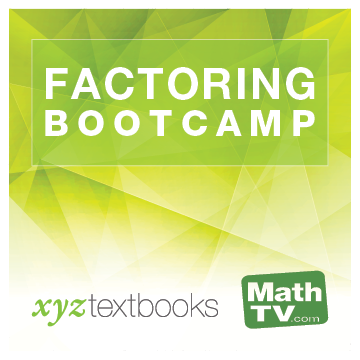#### Videos for:Factoring Bootcamp
Reducing Rational Expressions to Lowest Terms

Select an Instructor to Play Video

Example 7
A solution of hydrochloric acid (HCl) and water contains 49 milliliters of water and 21 milliliters of HCl. Find the ratio of HCl to water and of HCl to the total volume of the solution.
Example 8
The Forest chair lift at the Northstar ski resort in Lake Tahoe is 5,603 feet long. If a ride on the lift takes 11 minutes, what is the average speed of the lift in feet per minute?

Mini Lecture
Reduce to lowest terms.

1. $$\displaystyle\frac{10a+20}{5a+10}$$

2. $$\displaystyle\frac{x^3+3x^2-4x}{x^3-16x}$$

3. $$\displaystyle\frac{4x^2-12x+9}{4x^2-9}$$Innovative products, peer tutors, fair prices, and unlimited access
www.xyztextbooks.com | info@mathtv.com